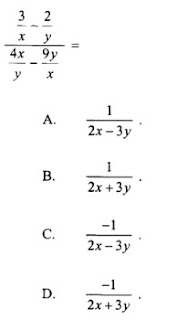## 程度 初階 中階 高階  技巧 (一) : 答案逐個試 (sub answer)  這技巧是用來check 數的(主要作用).把每一個答案sub入題目中，直到找到答案。   Example 1   Let a and b be non-zero numbers. If 7a + 5b = 3a + 8b, then a : b =設 a 和 b 為非零整數。如果7a + 5b = 3a + 8b, 然後a : b =A.       3 : 4B.        4 : 3C.        10 : 13D.        13 : 10A.       7(3) + 5(4) = 41          3(3) + 8(4) = 41B.        7(4) + 5(3) = 43          3(4) + 8(3) = 36C.        7(10) + 5(13) = 1353(10) + 8(13) = 134D.        7(13) + 5(10) = 141        3(13) + 8(10) = 119所以答案是A.(adsbygoogle = window.adsbygoogle || []).push({});    Try youself答案 D  (反白)  技巧 (二) : 自定變數(sub variable)  這技巧是用來增快計數的速度。自己在題目中沒有設下的變數，設下一些數值。   Example 1Let x = 5   y = 7ANS = -0.032258經過計算A B C D，便可得出答案：D注意：當做這類題目，一些題目中沒有的數字，以免令自己誤會。   Try youself 1答案：(反白下面)Dlet A = 30Ans = 2.309   Try youself 2答案 (反白下面)let θ = 60ans = 0.3360答案：D  特別注意  有關 sin cos tan 的題目，多數可以用這種技巧來做。唯一要注意的是：請適當設 sin cos tan 中的 variable,盡量使 sin cos tan 出來的數值不是零.多數是設degree 30/60,不要設為45，因為sin45=cos45，這有時會玩死自己的。(adsbygoogle = window.adsbygoogle || []).push({}); 初階中階高階

1.2.